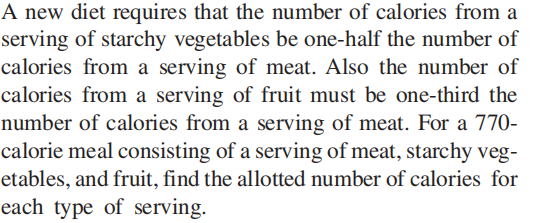### Still have math questions?

Algebra
Question$$\left. \begin{array} { l } { \text { A new diet requires that the number of calories from a } } \\ { \text { serving of starchy vegetables be one- half the number of } } \\ { \text { calories from a serving of meat. Also the number of } } \\ { \text { calories from a serving of fruit must be one- third the } } \\ { \text { number of calories from a serving of meat. For a } 770 \text { - } } \\ { \text { calorie meal consisting of a serving of meat, starchy veg- } } \\ { \text { etables, and fruit, find the allotted number of calories for } } \\ { \text { each type of serving. } } \end{array} \right.$$

$$\text { Meat } 420 \text { calories; vegetable } 210 \text { calories; fruit } 140 \text { calories }$$